American Journal of Physics and Applications
Volume 3, Issue 6, November 2015, Pages: 221-225

New Approach to Geometrization of Electromagnetic Field

O. A. Olkhov

Department of Theoretical Physics, Institute of Chemical Physics, Moscow, Russia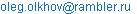O. A. Olkhov. New Approach to Geometrization of Electromagnetic Field. American Journal of Physics and Applications. Vol. 3, No. 6, 2015, pp. 221-225. doi: 10.11648/j.ajpa.20150306.16

Abstract: The new approach to geometrization of electromagnetic field is suggested, where previous author’s results on geometrical interpretation of quantum objects are taken into account. These results can be considered as a justification for considering of spaces with higher dimensions for geometrization of electromagnetic field. Electromagnetic fields and potentials are considered here as components of torsion tensor in 5-dimensional affinely connected space where the usual 4-space-time is a pseudo-Euclidean hyperplane. Electromagnetic potentials and tensor of electromagnetic field are represented by different components of the torsion tensor as it should be for the notions of different physical meaning. Suggested geometrization is free of such disadvantages of the known 5-dimensional Kaluza’s theory as the absence of physical foundations for introduction of additional spatial dimensions and the lack of any relationship with quantum mechanics.

Keywords: Geometrical Paradigm, Geometrical Interpretation of Quantum Mechanics, Geometrization of Electromagnetic Field

Contents

1. Introduction

Geometrization of electromagnetic field is one of the problems on the way of establishing the geometrical paradigm in physics where all mater is considered as some deformation of the space. This idea was suggested in 19 century by mathematicians Clifford and Riemann, and the first confirmation of this idea was obtained by Einstein who showed that in general relativity gravitational field can be interpreted as some distortion of the space geometry. Soon after this attempt investigations of geometrization of the electromagnetic field was undertaken by Einstein himself and by many of his followers. At first this work was directed to finding such non-Euclidean geometry of physical 4-space whose geometrical characteristics could be identified with electromagnetic fields and potentials, but all attempts on this way have failed. Another approach was proposed by Kaluza who assumed that geometrical meaning of electromagnetism can be connected with geometrical properties of 5-dimensional Riemannian space-time . In particular, he identified electromagnetic potentials with some components of the metric tensor of this space. However, this concept met many objections. As its main disadvantages Einstein indicated the absence of physical prerequisites for introducing fifth dimension and the absence of any connection with quantum mechanics . Subsequent attempts to geometrize electromagnetic fields had no success, and the interest in the problem for the rest of 20th century essentially decreased (though there were attempts to use the idea of many-dimensional spaces for developing unified theory of all known physical fields  and there were rare attempts to introduce geometrical ideas in physics within the framework of the usual 4-dimensional space-time ).

In this work the geometrization of electromagnetic field is achieved via 5-dimensional approach that is free from above-mentioned disadvantages. The principally new idea here is that we use as the starting point the geometrization of quantum objects, suggested earlier by the author [4-9]. This geometrization assumes that quantum particles can be considered as projections of the spatial topological defects moving in the space with dimensionality more than three. We show that electromagnetic fields and potentials can be represented as different components of torsion tensor of the 5-dimentional affinely-connected space where the usual 4-space-time is a pseudo-Euclidean hyperplane. Notice that different geometrical representation of fields and potentials is in line with their different physical nature demonstrated, in particular, by Aharonov-Bohm effect .

This geometrization of electromagnetic field is the geometrization of the bose-field. Geometrization of the Dirac equation, suggested earlier by the author, can be considered as geometrization of the fermi-field, and we start with presentation of the main points of this geometrization [4-9].

2. Geometrization of Quantum Particles

Let us remind at first the main points of the suggested geometrical interpretation of quantum mechanics. The starting moment here is the topological interpretation of the Dirac’s relativistic equation for free particle with spin ½. Symmetric form of this equation is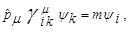(1)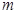is the mass of the particle,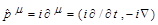,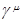(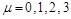) are 4x4 Dirac’s matrices,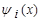- Dirac’s byspinor,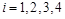. We use in (1) the relativistic units where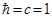. Here and later on we use the 4-metrics with signature (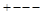). With such units the elementary charge square is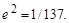Solution of Eq.(1) for free particle’s states with definite values of 4-momentum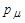has the form of the plane wave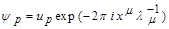(2)

where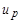-- definitely normalized byspinor and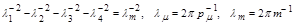(3)

Within traditional interpretation Eq. (1) describes the only possible results of measurements over quantum object, namely possible values of its 4-momentum. But this equation does not include any other information about the object before or between the measurements (what it is within classical notions, for example). However in our hypothesis we assume that Eq. (1) inсludes in fact such information. The starting point is the fact that above solution of Eq. (1) can be interpreted as a basic vector of a representation for the infinity translation group, including all translations of the form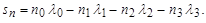(4)

Here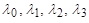- four basic orthogonal vectors into four - dimensional pseudoeuclidean space and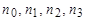- integers. The physical pseudo-Euclidean space-time does not have such symmetry (corresponding to a symmetry of infinite сrystal), and our main suggestion is that this group acts in the auxiliary space--the universal covering space of some closed 4-manifold described by Eq. (1). Such spaces are used in topology for description of closed manifolds because discrete groups, working into such spaces, isomorphic to so called manifold’s fundamental groups. Elements of this group are different classes of closed paths that starts and finishes at the same point (Poincare group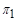[12-14]). In particular, an infinite translation group operating in one-dimensional Euclidian space is isomorphic to a fundamental group of the one-dimensional closed manifold homeomorphic to a circle. An infinite translation group operating in two-dimensional Euclidean space is isomorphic to a fundamental group of the two-dimensional closed manifold homeomorphic to a torus [12-14]. In addition, the wave function (3) is a byspinor—tensor realizing two-valued representation of a rotation group and so can be considered as a description of a symmetry of nonorientable geometrical objects [15,16].

This consideration leads to a hypothesis that (1) can be considered as a description of a closed manifold (by coordinates of its universal covering space), namely, as a description of the closed nonorientable topological space-time 4-manifold, where spin ½ corresponds to index of the two-valued rotation group. It can be shown that due to the pseudo-Euclidean metric of the physical space-time such four-dimensional object represents moving microscopic region of three-dimensional space of size of order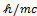and that this region is an intersection with the topological defect that is located in external space of higher dimensionality. Such geometrical construction has wave-corpuscular and stochastic properties. This gives an opportunity to identify this geometrical object with the quantum object described by Eq. (1) [4-7]. It was also shown that application of this interpretation to the atomic spectra theory leads to reasonable results in calculations of spectrum of a helium atom [8,9].

The fact that geometrical representation of quantum objects needs introduction of spaces of higher dimensionality plays, as we will see, a principal role in geometrization of classical electromagnetic field. Therefore, let us remind how closed 4-space-time manifold can represent movement of topological defect of three-dimensional space in the space of higher dimensionality. We use now the low dimensional analogy. We have to notice that modern 4-dimensional topology does not give any opportunity to discuss in detail the properties of 4-dimensional manifolds (in any case there is even no classification of 4-manifolds [12,13]). Therefore the low dimensional analogy is the only possibility to demonstrate specific features of manifolds representing quantum particles. Let us consider the simplest low dimensional realization of our 4-space-time --- 2-dimensional pseudo Euclidean space-time, whose inhabitants are one dimensional creatures living in one dimensional Euclidean space. Let us consider one of the simplest closed space-time topological manifolds that is possible in this space --- two dimensional manifold, homeomorphic to a torus (such manifold represents quantum particle in this space). And, at last, let us consider the usual torus without all his possible homeomorphisms. (Such torus is specified by topological product of two circles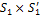). In Euclidean space this torus looks as known closed surface, represented at Fig. 1A.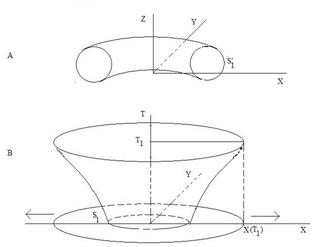Figure 1. Geometrization of the free fermi-field (low-dimensional analogy).

In pseudo Euclidean space one of the circles (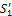) is turned to hyperbola , and our torus looks as a hyperboloid (Fig. 1B). Cross-sections of this hyperboloid for different values of time by planes parallel to XY-plane describe movement in this plane of expanding circle. The habitants of one dimensional world could only detect the points of intersection X (T) of expanding circle with their world and they will interpret these points as moving quantum particles. As for circle itself, it will be considered as topological defect of the "physical" one dimensional space situated in external two dimensional space.

3. Geometrization of Classical Electromagnetic Field

Let us underline the special features of geometrical representation of quantum particles that have to be taken into account in geometrization of the electromagnetic field.

1) Geometrical representation of quantum particle needs introduction of the "external" space with dimensionality more than three (aside from time-dimension).

2) Topological defect corresponding to quantum particle occupies a finite region of microscopic size in the "external" space.

These comments lead to the suggestion that the geometrical representation of electromagnetic field also has to include consideration of external 5-dimensional space where physical space-time is a 4-dimensional pseudo Euclidean hyperplane. This external space is closed and runs in the direction of fifth dimension to а microscopic distance (of order of atomic sizes).

It is easy to see that the starting point coincides with the idea of the known work of Kaluza  where he firstly suggested to consider fifth dimension for the geometrization of electromagnetic field. And the hypothesis about closed space in the direction of fifth dimension discussed earlier by Einstein and Bergmann . But there were no any quantum mechanical foundations in both these works for suggested hypotheses, and here is the first essential distinction from our work. And the second main distinction is in choosing of the geometry of the external 5-dimensional space. It was suggested earlier that 5-dimensional space is a Riemannian one, and then some components of Riemannian metric were identified with components of electromagnetic potential. Such approach have not been successful (the history of the problem can be seen in ). Geometrical interpretation of quantum particles, suggested by the author, considers these particles as topological manifolds that have no definite form. This indicates that geometry of 5-dimensional space (within geometrization of electromagnetic field) should be more complex than Riemmanian one – a simplest of non Euclidean geometries. Therefore, in this work we suggest that electromagnetic field should be identified with geometrical properties of non Riemannian space, namely, with properties of affinely connected space with torsion where the usual 4-space-time is a pseudo-Euclidean hyperplane.

Let us recall the distinctive features of affinely connected space with torsion . In affinely connected space the field of so called affine connectedness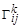is preassigned on manifold for every coordinate system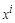, and this connectedness is transformed with coordinate transformations by the law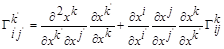(5)

The torsion tensor of this space is defined as antisymmetric tensor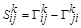and this tensor is different from zero in our case. Its geometrical meaning is that it violates the parallelogram rule .

Geometrization of electromagnetic field is the establishing of correspondence between components of torsion tensor and characteristics of this field. Indexes of a torsion tensor take five different values in correspondence with five coordinates of the space being considered. Each component of the torsion tensor depends in general on five coordinates – four coordinates of the pseudo Euclidean physical space-time (that is hyperplane in 5-dimension space) and on fifth coordinate. In accordance with remarks at the beginning of this Section we suggests that above 5-dimensional space is closed in the direction of fifth dimension and that the space runs in this direction to a microscopic distance. The macroscopic scales are used in description of classical fields. Therefore we suggest that the dependence of the torsion tensor on fifth coordinate can be neglected.

We denote possible values of space-time coordinate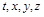by Greek letters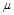,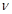or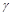and we denote index of fifth dimension in the torsion tensor by digit 5. Then nonzero components of the antisymmetric torsion tensor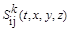can be written as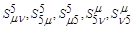(6)

First of all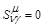because for 4-dimensional space-time torsion is zero. Componentshave the same tensor properties in the 4-space-time as components of antisymmetric tensor of electromagnetic field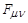and can be identified with this tensor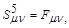(7)

and components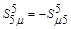have the same tensor proprieties in the 4-space=time as components of electromagnetic potentials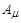and they can be identified with these 4-vectors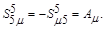(8)

As for "superfluous" components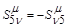, we have to take into account that electromagnetic interaction is always exists along with weak interaction forming the so called electroweak interaction . We suggest that above components are ‘traces" of weak interaction and that they can be neglected within consideration of electromagnetic interaction. Then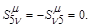(9)

Notice that relation (9) is relativistic invariant and, consequently, it is true in 4-space-time for any coordinate system.

According to (7, 8), components of tensor of electromagnetic field (electric and magnetic fields) and components of electromagnetic potential are defined by essentially different components of torsion tensor. This confirms the fact, demonstrated by Aharonov-Bohm effect, that electromagnetic potentials are not abstract mathematical values. useful for calculations of electromagnetic fields, but that they have their own physical meaning .

Finally, geometrization of electromagnetic field is defined by relations (7, 8). These relations establish correspondence between physical characteristics of electromagnetic field in pseudo Euclidean 4-space-time and geometrical characteristics of 5-dimensional non Euclidean space.

4. Results

The main results are:

1.  It is shown that a necessity of consideration of 5-dimensional space-time in geometrization of classical electromagnetic field is a consequence of necessity of consideration of 5-dimensional space-time in geometrization of quantum particles.

2.  It is supposed that in geometrization of electromagnetic field the above 5-dimensional space is a affine space with torsion where physical 4-space-time is a hyperplane.

3.  The relations established between components of the field and components of the potential and principally different components of torsion tensor of above 5-dimensional space (this can be considered as geometrical interpretation of effect of Aharonov-Bohm).

5. Conclusion

The results obtained in the paper is the first (known to the author) attempt to geometrize bose- and fermi-fields simultaneously. Because such fields are in foundation of all matter these results may be important from the point of view of establishing of geometrical paradigm. There are of course many other problems to be solved: geometrization of other gauge fields, geometrization of quantized fields, and so on. Some of these problems will be considered in subsequent publications.

Acknowledgements

The author is grateful to Garnik G. Aleksanyan and G. V. Golubkov for their support.

References

1. Th. Kaluza, "On the Unification Problem in Physics," Sitzungsberichte Pruss. Acad. Sci, pp.966-972, 1921.
2. A. Einstein and P. Bergmann, "On A Generalization of Kaluza’s Theory of Electricity", Annals of Mathematics 39 p.685, 1938.
3. Y.S. Vladimirov, Geometrophysics (in Russian). Moscow, Binom, 2005.
4. O. A. Olkhov. "Geometrization of Quantum Mechanics," Journal of Phys.: Conf.Ser. vol.67, p.012037. 2007.
5. O. A. Olkhov. "Geometrization of Classical Wave fields," Mellwill, New York, AIP Conference Proceedings p.316, vol.962,2008(Proc.Int.Conf. "Quantum Theory: Reconsideration of Foundations." Vaxje, Sweden. 11-16 June,2007).
6. O. A. Olkhov, "Geometrization of matter wave fields and electromagnetic waves," Proc.of X111 Int.Scientific Meeting PIRT—2007, p.318, Moscow; 2-5 July, 2007. Moscow-Liverpool-Sanderland.
7. O. A. Olkhov, "On the possibility of topological interpretation of quantum mecanics", arXiv: 0802.2269, 2008.
8. O. A. Olkhov. "Geometrical approach to the atomic spectra theory. The helium atom," Russian J. of Phys.Chemistry B, vol.8, pp.30---42, February 2014[Chim,Fis, p.36, vol.33, №2, 2014].
9. Oleg Olkhov. "Geometrical approach in atomic physics: Atoms of hydrogen and helium," American Journal of Physics and Applications, vol.2, №5, pp.108---113, 2014.
10. Y. Aharonov and D. Bohm, "Significance of electromagnetic potentials in quantum theory," Phys.Rev, vol.115, pp.485---491, 1959.
11. L. D. Landau, E. M. Lifshitz, Course of Theoretical Physics. Vol.4; V. B. Berestetzki, E. M. Lifshitz, L. P. Pitaevski, Quantum Electrodynamics, Butterworth-Heinemann, 1982.
12. B. A. Dubrovin, A. T. Fomenko, S. P. Novikov, Modern geometry---Methods and Applications, Part 2: The Geometry and Topology of Manifolds, Springer, 1985.
13. A. S. Schwartz, Quantum field theory and topology, Grundlehren der Math.Wissen.307, Springer, 1993.
14. H.S. M. Coxeter, Introduction to geometry, John Wiley & Sons, New York, London,1961.
15. P. K. Rachevski. Riemannian geometry and tensor analisis (in Russian), Moscow, Nauka, 1966.
16. V.A. Jelnorovitch Theory of spinors and its applications (in Russian), Moscow, August-Print, 2001.
17. Ta-Pei Cheng, Ling-Fong Li, Gauge theory of elementary particle physics, Oxford, Clarendon Press, 1984.
18. Sharon Eitan. "Towards a geometrical foundation for physics," SOP Transaction on Theoretical Physics, vol.2, №1,pp.89---129, 2015.

 Contents 1. 2. 3. 4. 5.
Article ToolsAbstractPDF(289K)